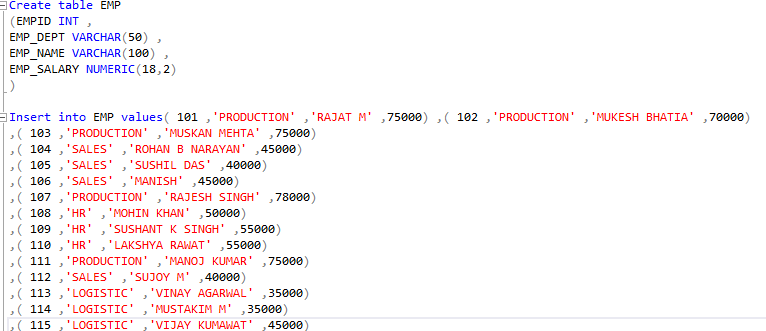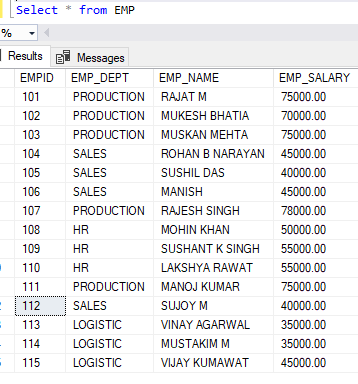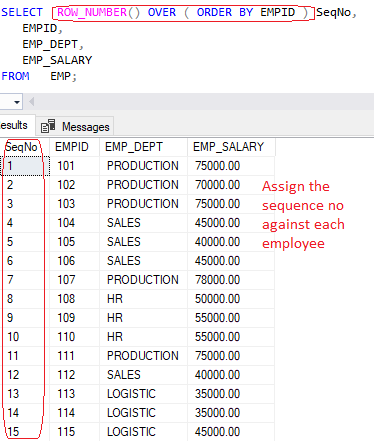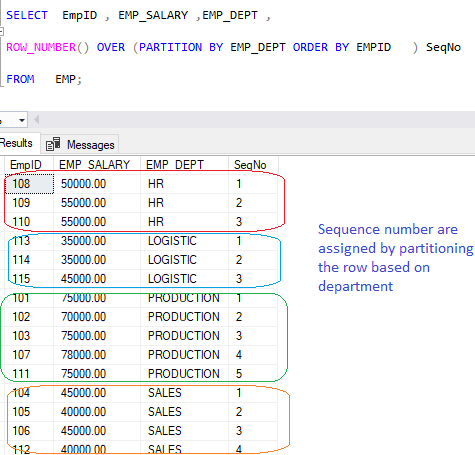Home » SQL Server ROW_NUMBER()

# SQL Server ROW_NUMBER()### SQL Row_Number() is a ranking function/window function that is used to assign a sequential integer to each row within a partition of a result set. The row number starts with one for the first row in each partition.

Syntax

```Select ROW_NUMBER() OVER (

[PARTITION BY colnames, ... ]

ORDER BY colnames [ASC | DESC]

) , col1 ,..

From table```

PARTITION BY

The PARTITION BY clause divides the result set into partitions.

Row_Number() function is applied to each partition separately and reinitialized the row number for each partition.

The PARTITION BY clause is optional. If we do not use , the Row_Number() function will consider the whole result set as a single partition.

ORDER BY

The ORDER BY clause defines the logical order of the rows within each partition of the result set.

The ORDER BY clause is mandatory because the Row_Number() function is order sensitive.

Lets Look at an example of Row_Number() in SQL .
First we create a table – EMP, having columns as Department , salary and Employee Name
```Create table EMP
(EMPID INT ,
EMP_DEPT VARCHAR(50) ,
EMP_NAME VARCHAR(100) ,
EMP_SALARY NUMERIC(18,2)
)

Insert into EMP values( 101 ,'PRODUCTION' ,'RAJAT M' ,75000) ,
( 102 ,'PRODUCTION' ,'MUKESH BHATIA' ,70000)
,( 103 ,'PRODUCTION' ,'MUSKAN MEHTA' ,75000)
,( 104 ,'SALES' ,'ROHAN B NARAYAN' ,45000)
,( 105 ,'SALES' ,'SUSHIL DAS' ,40000)
,( 106 ,'SALES' ,'MANISH' ,45000)
,( 107 ,'PRODUCTION' ,'RAJESH SINGH' ,78000)
,( 108 ,'HR' ,'MOHIN KHAN' ,50000)
,( 109 ,'HR' ,'SUSHANT K SINGH' ,55000)
,( 110 ,'HR' ,'LAKSHYA RAWAT' ,55000)
,( 111 ,'PRODUCTION' ,'MANOJ KUMAR' ,75000)
,( 112 ,'SALES' ,'SUJOY M' ,40000)
,( 113 ,'LOGISTIC' ,'VINAY AGARWAL' ,35000)
,( 114 ,'LOGISTIC' ,'MUSTAKIM M' ,35000)
,( 115 ,'LOGISTIC' ,'VIJAY KUMAWAT' ,45000)

```Now we have a EMP table, Lets select the records from table.
`select * from EMP`#### Generate Sequential number using ROW_NUMBER()

To assign the sequence number to each employee in Emp table, you can use the Row_Number() function as given below.
```SELECT ROW_NUMBER() OVER ( ORDER BY EMPID ) SeqNo,
EMPID,
EMP_DEPT,
EMP_SALARY
FROM EMP;```#### Row_number() with Partition By

Following statement uses Row_Number() function to assign a sequence number by partitioning the row based on department Name.
```SELECT EmpID , EMP_SALARY ,EMP_DEPT ,

ROW_NUMBER() OVER (PARTITION BY EMP_DEPT ORDER BY EMPID ) SeqNo

FROM EMP;```Lets take one more example using Row_Number() with partition by
Assign sequence by partitioning the employees based on department and salary, and Sequence number order will be based on salary in descending order.
```SELECT EmpID ,EMP_DEPT , EMP_SALARY,

ROW_NUMBER()OVER(PARTITION BY EMP_DEPT, EMP_SALARY ORDER BY EMP_SALARY desc )SeqNo

FROM EMP;```